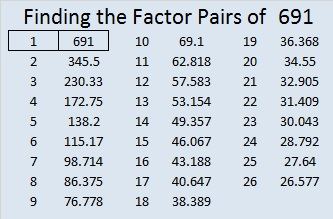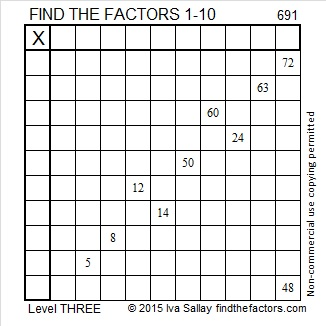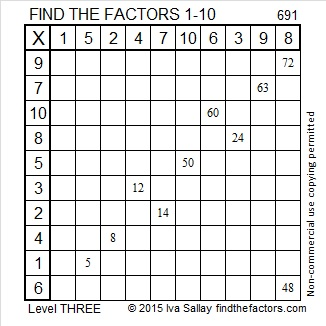# 691 and Level 3

• 691 is a prime number.
• Prime factorization: 691 is prime.
• The exponent of prime number 691 is 1. Adding 1 to that exponent we get (1 + 1) = 2. Therefore 691 has exactly 2 factors.
• Factors of 691: 1, 691
• Factor pairs: 691 = 1 x 691
• 691 has no square factors that allow its square root to be simplified. √691 ≈ 26.2868789.How do we know that 691 is a prime number? If 691 were not a prime number, then it would be divisible by at least one prime number less than or equal to √691 ≈ 26.3. Since 691 cannot be divided evenly by 2, 3, 5, 7, 11, 13, 17, 19, or 23, we know that 691 is a prime number.

Here is today’s puzzle:Print the puzzles or type the solution on this excel file: 10 Factors 2015-11-23

———————————————————————————

Here’s a little more about 691:

691 is the sum of the thirteen prime numbers from 29 to 79.

691 is equivalent to palindrome 171 in BASE 23. Note that 1(23²) + 7(23) + 1(1) = 691.

———————————————————————————

A Logical Approach to solve a FIND THE FACTORS puzzle: Find the column or row with two clues and find their common factor. (None of the factors are greater than 10.)  Write the corresponding factors in the factor column (1st column) and factor row (top row).  Because this is a level three puzzle, you have now written a factor at the top of the factor column. Continue to work from the top of the factor column to the bottom, finding factors and filling in the factor column and the factor row one cell at a time as you go.This site uses Akismet to reduce spam. Learn how your comment data is processed.